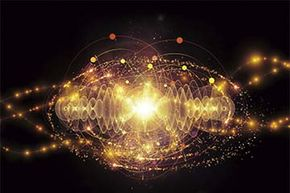# How much does light weigh?

By: Kate Kershner  |Does light weigh anything? Well, yes and no.
agsandrew/iStock/Thinkstock

If there were a simple answer to how much light weighs, we'd all know it. There would probably be some sort of elementary school rhyme to help us remember the exact figure, much like our useful little ditty about 1492 and the ocean blue.

Instead, we are forced to wade through complicated half-answers that go something like, "Um, it kind of weighs a little, but not like how regular things weigh." Which is a terrible jingle to sing on the playground.

Let's try to un-muddle the mess and start by asking a different question altogether: Do photons have mass?

Photons are the smallest measure of light, and no, they don't have mass. So that's easy, right? Light is composed of photons, which have no mass, so therefore light has no mass and can't weigh anything.

Not so fast. Because photons have energy -- and, as Einstein taught us, energy is equal to the mass of a body, multiplied by the speed of light squared. How can photons have energy if they have no mass? (One imagines Einstein thinking about photons re: mass and shrugging, hoping that nobody noticed the discrepancy.)

Actually, what Einstein was proving is that energy and mass could be the same thing -- all energy has some form of mass. Light may not have rest (or invariant) mass -- the weight that describes the heft of an object. But because of Einstein's theory (and the fact that lightbehaves like it has mass, in that it's subject to gravity), we can say that mass and energy exist together. In that case, we'd call it relativistic mass -- mass when an object is in motion, as opposed to at rest [source: Gibbs].

So our answer is a grab bag of yeses and nos. Does light have a mass that can be weighed on the bathroom scale? Most certainly not. But it is a source of gravitational fields, so we could say that a box of light weighs more than a box without light -- as long as you're comfortable understanding that the "weight" you're measuring is a form of energy and not, say, pounds or kilograms [source: Ask the Van].

Originally Published: Sep 22, 2014

### Light Weight FAQ

##### Does light have weight?
Sort of. All energy has some form of mass and light is no exception. So light has weight, but not the kind of mass you can weigh on a bathroom scale.
##### What is mass of light?
There’s no clearcut answer to this. Light is formed by photons that move around to form the brightness we see. Photons themselves have no mass of their own, but they have energy when they move, which Einstein said could be the same thing.
##### Does gravity affect things without mass?
Gravity impacts almost everything that carries energy, even a particle without any mass. This is why the gravitational energy of dark matter can change the path of light in space.
##### Why is light affected by gravity?
Gravity is the force that bends light and curves it inwards or outwards. Light dips and curves when it faces massive objects, known as gravitational lensing.
##### What is the weight of electricity?
If you consider electricity in the form of the electrons that make it up, it does weigh a tiny bit. The weight is insignificant though, around a gram for 1000,000,000 joules rated at one volt.

### Author's Note: How much does light weigh?

Understanding the relationship between light and mass and photons and energy is daunting. It's enough to make one wish Einstein could patiently walk us through it himself.

### Sources

• Ask the Van. "How does light have momentum without mass?" University of Illinois at Urban-Champaign Department of Physics. 2014. (June 25, 2014) http://van.physics.illinois.edu/qa/listing.php?id=1424
• Ask the Van. "Light's Weight." University of Illinois at Urbana-Champaign Department of Physics. 2014. (June 25, 2014) http://van.physics.illinois.edu/qa/listing.php?id=1395
• Ask the Van. "Photons, mass, gravity, light, rest mass, invariant mass, energy, momentum." University of Illinois at Urban-Champaign Department of Physics. 2014. (June 25, 2014) http://van.physics.illinois.edu/qa/listing.php?id=16351
• Gibbs, Philip, Carr, Jim and Koks, Don. et al. "What is relativistic mass?" Physics and Relativity FAQ. 2012. (June 25, 2014) http://math.ucr.edu/home/baez/physics/Relativity/SR/mass.html
• Gibbs, Philip. "Does light have mass?" Physics and Relativity FAQ . 1997. (June 25, 2014) http://www.desy.de/user/projects/Physics/Relativity/SR/light_mass.html
• Newton. "Sunlight and weight." Argonne National Laboratory. June 2012. (June 25, 2014) http://www.newton.dep.anl.gov/askasci/phy00/phy00644.htm
• UCSB ScienceLine. "I was wondering if light...." University of California Santa Barbara. (June 25, 2014) http://scienceline.ucsb.edu/getkey.php?key=2976